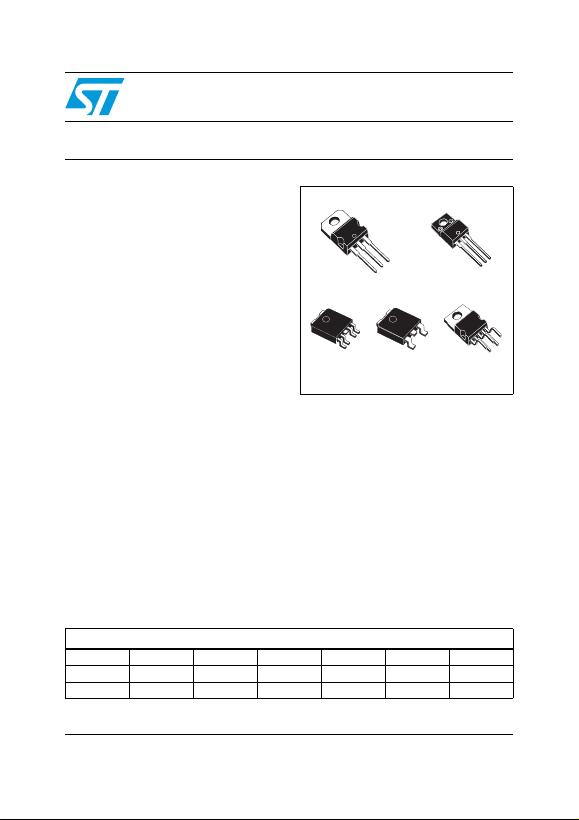February 2012 Doc ID 2574 Rev 25 1/50
50
LFxxAB
LFxxC
Very low drop voltage regulators with inhibit
Features
Very low dropout voltage (0.45 V)
Very low quiescent current (typ. 50 µA in OFF
mode, 500 µA in ON mode)
Output current up to 500 mA
Logic-controlled electronic shutdown
Output voltages of 1.5; 1.8; 2.5; 3.3; 4.7; 5; 6;
8; 8.5; 9; 12 V
Automotive Grade product: 1.8 V, 2.5 V, 3.3 V,
5.0 V, 8.0 V, 8.5 V VOUT in DPAK and PPAK
packages
Internal current and thermal limit
Only 2.2 µF for stability
Available in ± 1 % (AB) or ± 2 % (C) selection
at 25 °C
Supply voltage rejection: 80 db (typ.)
Temperature range: -40 to 125 °C
Description
The LFxxAB/LFxxC are very low drop regulators
available in PENTAWATT, TO-220, TO-220FP,
DPAK and PPAK package and in a wide range of
output voltages. The very low drop voltage (0.45
V) and the very low quiescent current make them
particularly suitable for low noise, low power
applications and specially in battery powered
systems. In the 5 pins configuration (PENTAWATT
and PPAK) a shutdown logic control function is
available (pin 2, TTL compatible). This means that
when the device is used as a local regulator, it is
possible to put a part of the board in standby,
decreasing the total power consumption. In the
three terminal configuration the device has the
same electrical performance, but is fixed in the
ON state. It requires only a 2.2 µF capacitor for
stability allowing space and cost saving. The LFxx
is available as Automotive Grade in DPAK and
PPAK packages, for the options of output voltages
whose commercial part numbers are shown in the
order codes. These devices are qualified
according to the specification AEC-Q100 of the
Automotive market, in the temperature range - 40
°C to 125 °C, and the statistical tests PAT, SYL,
SBL are performed.
TO-220FP
PENTAWATT
TO-220
DPAKPPAK
Table 1. Device summary
Part numbers
LF15AB LF25C LF33AB LF60C LF80AB LF90C LF120AB
LF18C LF25AB LF50C LF60AB LF85C LF90AB
LF18AB LF33C LF50AB LF80C LF85AB LF120C
www.st.comContents LFxxAB, LFxxC
2/50 Doc ID 2574 Rev 25
Contents
1 Diagram . . . . . . . . . . . . . . . . . . . . . . . . . . . . . . . . . . . . . . . . . . . . . . . . . . . 5
2 Pin configuration . . . . . . . . . . . . . . . . . . . . . . . . . . . . . . . . . . . . . . . . . . . 6
3 Maximum ratings . . . . . . . . . . . . . . . . . . . . . . . . . . . . . . . . . . . . . . . . . . . . 7
4 Electrical characteristics . . . . . . . . . . . . . . . . . . . . . . . . . . . . . . . . . . . . . 8
5 Typical performance characteristics . . . . . . . . . . . . . . . . . . . . . . . . . . . 35
6 Package mechanical data . . . . . . . . . . . . . . . . . . . . . . . . . . . . . . . . . . . . 39
7 Order codes . . . . . . . . . . . . . . . . . . . . . . . . . . . . . . . . . . . . . . . . . . . . . . . 48
8 Revision history . . . . . . . . . . . . . . . . . . . . . . . . . . . . . . . . . . . . . . . . . . . 49LFxxAB, LFxxC List of tables
Doc ID 2574 Rev 25 3/50
List of tables
Table 1. Device summary . . . . . . . . . . . . . . . . . . . . . . . . . . . . . . . . . . . . . . . . . . . . . . . . . . . . . . . . . . 1
Table 2. Absolute maximum ratings . . . . . . . . . . . . . . . . . . . . . . . . . . . . . . . . . . . . . . . . . . . . . . . . . . 7
Table 3. Thermal data. . . . . . . . . . . . . . . . . . . . . . . . . . . . . . . . . . . . . . . . . . . . . . . . . . . . . . . . . . . . . 7
Table 4. Electrical characteristics for LF15AB . . . . . . . . . . . . . . . . . . . . . . . . . . . . . . . . . . . . . . . . . . 8
Table 5. Electrical characteristics for LF18AB . . . . . . . . . . . . . . . . . . . . . . . . . . . . . . . . . . . . . . . . . . 9
Table 6. Electrical characteristics for LF18C . . . . . . . . . . . . . . . . . . . . . . . . . . . . . . . . . . . . . . . . . . 10
Table 7. Electrical characteristics for LF18CDT-TRY (Automotive Grade). . . . . . . . . . . . . . . . . . . . 11
Table 8. Electrical characteristics for LF25AB . . . . . . . . . . . . . . . . . . . . . . . . . . . . . . . . . . . . . . . . . 12
Table 9. Electrical characteristics for LF25ABDT-TRY (Automotive Grade). . . . . . . . . . . . . . . . . . . 13
Table 10. Electrical characteristics for LF25C . . . . . . . . . . . . . . . . . . . . . . . . . . . . . . . . . . . . . . . . . . 14
Table 11. Electrical characteristics for LF25CDT-TRY (Automotive Grade). . . . . . . . . . . . . . . . . . . . 15
Table 12. Electrical characteristics for LF33AB . . . . . . . . . . . . . . . . . . . . . . . . . . . . . . . . . . . . . . . . . 16
Table 13. Electrical characteristics for LF33C . . . . . . . . . . . . . . . . . . . . . . . . . . . . . . . . . . . . . . . . . . 17
Table 14. Electrical characteristics for LF33CDT-TRY and LF33CPT-TRY (Automotive Grade) . . . . 18
Table 15. Electrical characteristics for LF50AB . . . . . . . . . . . . . . . . . . . . . . . . . . . . . . . . . . . . . . . . . 19
Table 16. Electrical characteristics for LF50ABDT-TRY (Automotive Grade). . . . . . . . . . . . . . . . . . . 20
Table 17. Electrical characteristics for LF50C . . . . . . . . . . . . . . . . . . . . . . . . . . . . . . . . . . . . . . . . . . 21
Table 18. Electrical characteristics for LF50CDT-TRY and LF50CPT-TRY (Automotive Grade) . . . . 22
Table 19. Electrical characteristics for LF60AB . . . . . . . . . . . . . . . . . . . . . . . . . . . . . . . . . . . . . . . . . 23
Table 20. Electrical characteristics for LF60C . . . . . . . . . . . . . . . . . . . . . . . . . . . . . . . . . . . . . . . . . . 24
Table 21. Electrical characteristics for LF80AB . . . . . . . . . . . . . . . . . . . . . . . . . . . . . . . . . . . . . . . . . 25
Table 22. Electrical characteristics for LF80C . . . . . . . . . . . . . . . . . . . . . . . . . . . . . . . . . . . . . . . . . . 26
Table 23. Electrical characteristics for LF80CDT-TRY (Automotive Grade). . . . . . . . . . . . . . . . . . . . 27
Table 24. Electrical characteristics for LF85AB . . . . . . . . . . . . . . . . . . . . . . . . . . . . . . . . . . . . . . . . . 28
Table 25. Electrical characteristics for LF85C . . . . . . . . . . . . . . . . . . . . . . . . . . . . . . . . . . . . . . . . . . 29
Table 26. Electrical characteristics for LF85CDT-TRY and LF85CPT-TRY (Automotive Grade) . . . . 30
Table 27. Electrical characteristics for LF90AB . . . . . . . . . . . . . . . . . . . . . . . . . . . . . . . . . . . . . . . . . 31
Table 28. Electrical characteristics for LF90C . . . . . . . . . . . . . . . . . . . . . . . . . . . . . . . . . . . . . . . . . . 32
Table 29. Electrical characteristics for LF120AB . . . . . . . . . . . . . . . . . . . . . . . . . . . . . . . . . . . . . . . . 33
Table 30. Electrical characteristics for LF120C . . . . . . . . . . . . . . . . . . . . . . . . . . . . . . . . . . . . . . . . . 34
Table 31. TO-220 mechanical data . . . . . . . . . . . . . . . . . . . . . . . . . . . . . . . . . . . . . . . . . . . . . . . . . . 39
Table 32. Order codes . . . . . . . . . . . . . . . . . . . . . . . . . . . . . . . . . . . . . . . . . . . . . . . . . . . . . . . . . . . . 48
Table 33. Document revision history . . . . . . . . . . . . . . . . . . . . . . . . . . . . . . . . . . . . . . . . . . . . . . . . . 49List of figures LFxxAB, LFxxC
4/50 Doc ID 2574 Rev 25
List of figures
Figure 1. Block diagram . . . . . . . . . . . . . . . . . . . . . . . . . . . . . . . . . . . . . . . . . . . . . . . . . . . . . . . . . . . . 5
Figure 2. Pin connections (top view) . . . . . . . . . . . . . . . . . . . . . . . . . . . . . . . . . . . . . . . . . . . . . . . . . . 6
Figure 3. Test circuit . . . . . . . . . . . . . . . . . . . . . . . . . . . . . . . . . . . . . . . . . . . . . . . . . . . . . . . . . . . . . . 7
Figure 4. Dropout voltage vs. output current . . . . . . . . . . . . . . . . . . . . . . . . . . . . . . . . . . . . . . . . . . . 35
Figure 5. Dropout voltage vs. temperature . . . . . . . . . . . . . . . . . . . . . . . . . . . . . . . . . . . . . . . . . . . . 35
Figure 6. Supply current vs. input voltage . . . . . . . . . . . . . . . . . . . . . . . . . . . . . . . . . . . . . . . . . . . . . 35
Figure 7. Supply current vs. input voltage . . . . . . . . . . . . . . . . . . . . . . . . . . . . . . . . . . . . . . . . . . . . . 35
Figure 8. Short circuit current vs. input voltage . . . . . . . . . . . . . . . . . . . . . . . . . . . . . . . . . . . . . . . . . 35
Figure 9. Supply current vs. temperature. . . . . . . . . . . . . . . . . . . . . . . . . . . . . . . . . . . . . . . . . . . . . . 35
Figure 10. Logic controlled precision 3.3 / 5.0 V selectable output . . . . . . . . . . . . . . . . . . . . . . . . . . . 36
Figure 11. Sequential multi-output supply . . . . . . . . . . . . . . . . . . . . . . . . . . . . . . . . . . . . . . . . . . . . . . 36
Figure 12. Multiple supply with ON / OFF toggle switch . . . . . . . . . . . . . . . . . . . . . . . . . . . . . . . . . . . 37
Figure 13. Basic inhibit functions . . . . . . . . . . . . . . . . . . . . . . . . . . . . . . . . . . . . . . . . . . . . . . . . . . . . . 37
Figure 14. Delayed turn-on . . . . . . . . . . . . . . . . . . . . . . . . . . . . . . . . . . . . . . . . . . . . . . . . . . . . . . . . . 38
Figure 15. Low voltage bulb blinker . . . . . . . . . . . . . . . . . . . . . . . . . . . . . . . . . . . . . . . . . . . . . . . . . . . 38
Figure 16. Drawing dimension TO-220 (type STD-ST Dual Gauge) . . . . . . . . . . . . . . . . . . . . . . . . . . 40
Figure 17. Drawing dimension TO-220 (type STD-ST Single Gauge) . . . . . . . . . . . . . . . . . . . . . . . . . 41
Figure 18. Drawing dimension tube for TO-220 Dual Gauge (mm.) . . . . . . . . . . . . . . . . . . . . . . . . . . 42
Figure 19. Drawing dimension tube for TO-220 Single Gauge (mm.) . . . . . . . . . . . . . . . . . . . . . . . . . 42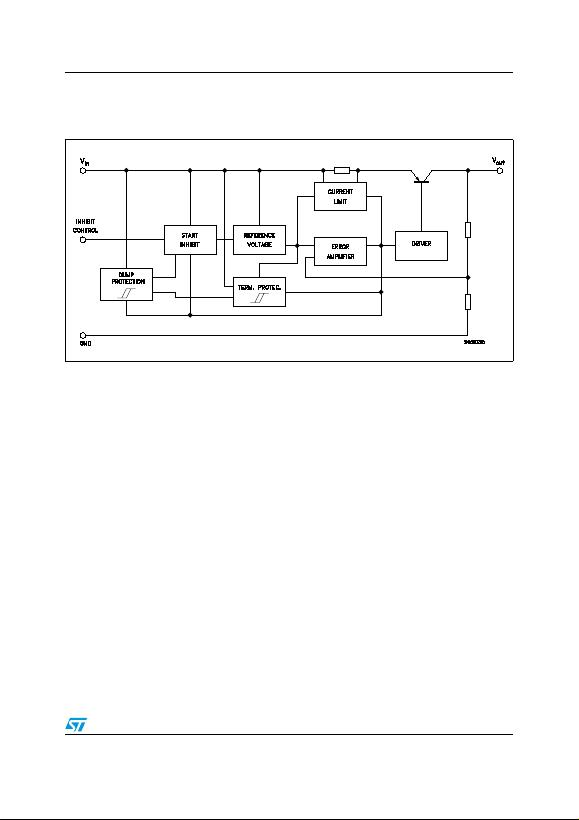LFxxAB, LFxxC Diagram
Doc ID 2574 Rev 25 5/50
1 Diagram
Figure 1. Block diagram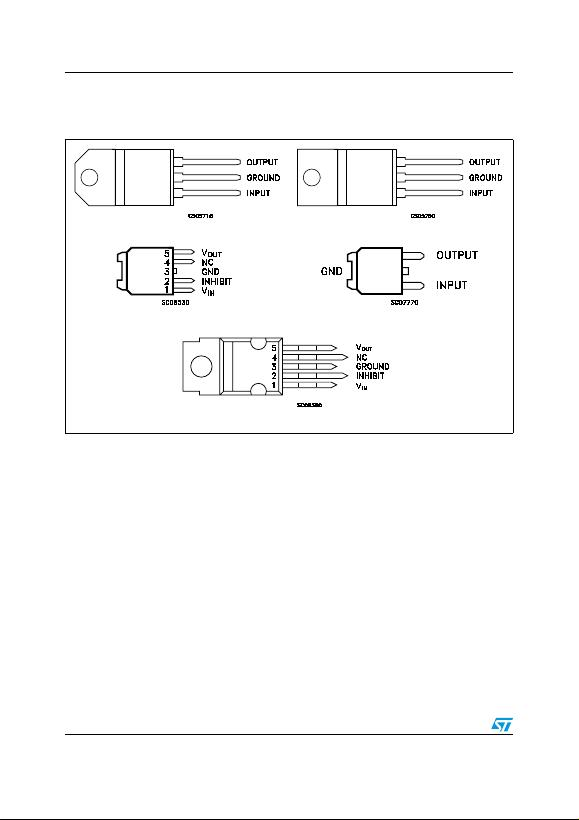Pin configuration LFxxAB, LFxxC
6/50 Doc ID 2574 Rev 25
2 Pin configuration
Figure 2. Pin connections (top view)
TO-220FP
DPAK
TO-220
PPAK
PENTAWATT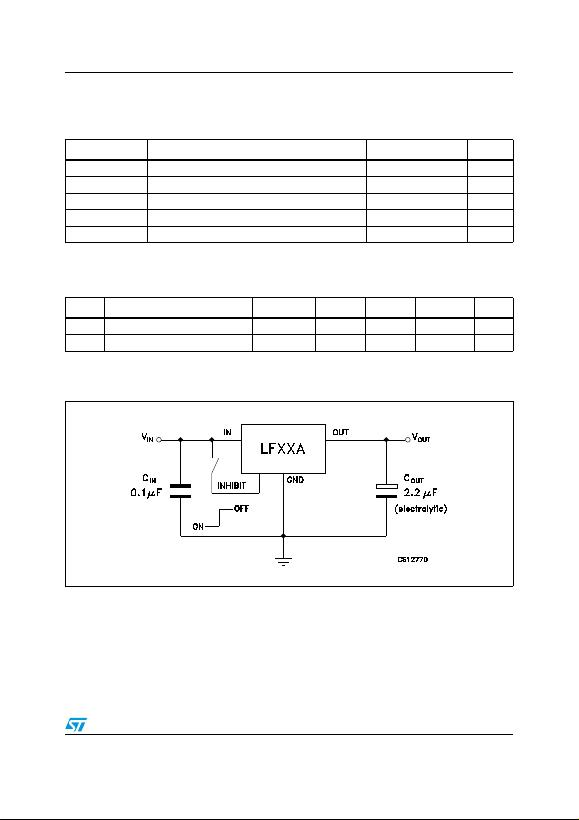LFxxAB, LFxxC Maximum ratings
Doc ID 2574 Rev 25 7/50
3 Maximum ratings
Table 2. Absolute maximum ratings
Symbol Parameter Value Unit
VIDC input voltage -0.5 to 40(1)
1. For 18 < VI < 40 the regulator is in shut-down
V
IOOutput current Internally limited
PTOT Power dissipation Internally limited
TSTG Storage temperature range -40 to 150 °C
TOP Operating junction temperature range -40 to 125 °C
Table 3. Thermal data
Symbol Parameter PENTAWATT TO-220 TO-220FP DPAK/PPAK Unit
RthJC Thermal resistance junction-case 3 5 5 8 °C/W
RthJA Thermal resistance junction-ambient 50 50 60 100 °C/W
Figure 3. Test circuit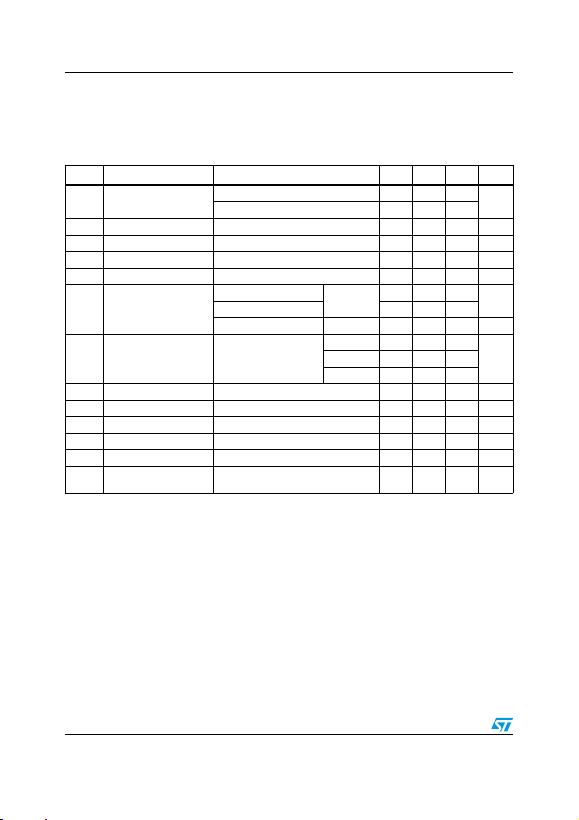Electrical characteristics LFxxAB, LFxxC
8/50 Doc ID 2574 Rev 25
4 Electrical characteristics
Refer to the test circuits, TJ = 25 °C, CI = 0.1 µF, CO = 2.2 µF unless otherwise specified.
Table 4. Electrical characteristics for LF15AB
Symbol Parameter Test conditions Min. Typ. Max. Unit
VOOutput voltage IO = 50 mA, VI = 3.5 V 1.485 1.5 1.515 V
IO = 50 mA, VI = 3.5 V, Ta = -25 to 85°C 1.470 1.530
VIOperating input voltage IO = 500 mA 2.5 16 V
IOOutput current limit 1 A
ΔVOLine regulation VI = 2.5 to 16 V, IO = 5 mA 2 10 mV
ΔVOLoad regulation VI = 2.8 V, IO = 5 to 500 mA 2 10 mV
IdQuiescent current
VI = 2.5 to 16V, IO = 0mA ON MODE 0.5 1 mA
VI = 2.8 to 16V, IO=500mA 12
VI = 6 V OFF MODE 50 100 µA
SVR Supply voltage rejection IO = 5 mA, VI = 3.5 ± 1 V
f = 120 Hz 82
dBf = 1 kHz 77
f = 10 kHz 65
eN Output noise voltage B = 10 Hz to 100 kHz 50 µV
VdDropout voltage IO = 200 mA 1 V
VIL Control input logic low Ta = -40 to 125°C 0.8 V
VIH Control input logic high Ta = -40 to 125°C 2 V
IIControl input current VI = 6 V, VC = 6 V 10 µA
CO
Output bypass
capacitance ESR = 0.1 to 10 Ω, IO = 0 to 500 mA 2 10 µF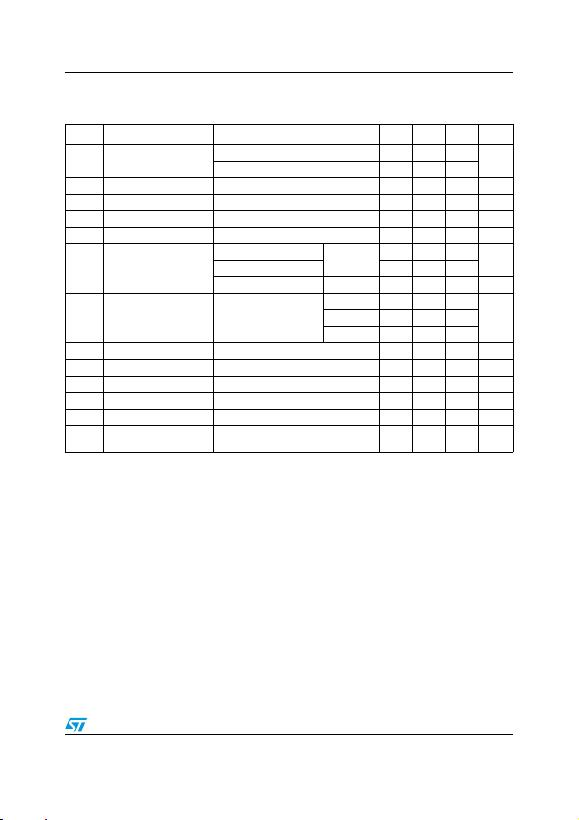LFxxAB, LFxxC Electrical characteristics
Doc ID 2574 Rev 25 9/50
Refer to the test circuits, TJ = 25 °C, CI = 0.1 µF, CO = 2.2 µF unless otherwise specified.
Table 5. Electrical characteristics for LF18AB
Symbol Parameter Test conditions Min. Typ. Max. Unit
VOOutput voltage IO = 50 mA, VI = 3.3 V 1.782 1.8 1.818 V
IO = 50 mA, VI = 3.3 V, Ta = -25 to 85°C 1.764 1.836
VIOperating input voltage IO = 500 mA 3 16 V
IOOutput current limit 1 A
ΔVOLine regulation VI = 2.8 to 16 V, IO = 5 mA 2 12 mV
ΔVOLoad regulation VI = 3.3 V, IO = 5 to 500 mA 2 10 mV
IdQuiescent current
VI = 2.5 to 16V, IO = 0mA ON MODE 0.5 1 mA
VI = 3.1 to 16V, IO=500mA 12
VI = 6 V OFF MODE 50 100 µA
SVR Supply voltage rejection IO = 5 mA, VI = 3.5 ± 1 V
f = 120 Hz 82
dBf = 1 kHz 77
f = 10 kHz 60
eN Output noise voltage B = 10 Hz to 100 kHz 50 µV
VdDropout voltage IO = 200 mA 0.7 V
VIL Control input logic low Ta = -40 to 125°C 0.8 V
VIH Control input logic high Ta = -40 to 125°C 2 V
IIControl input current VI = 6 V, VC = 6 V 10 µA
CO
Output bypass
capacitance ESR = 0.1 to 10 Ω, IO = 0 to 500 mA 2 10 µF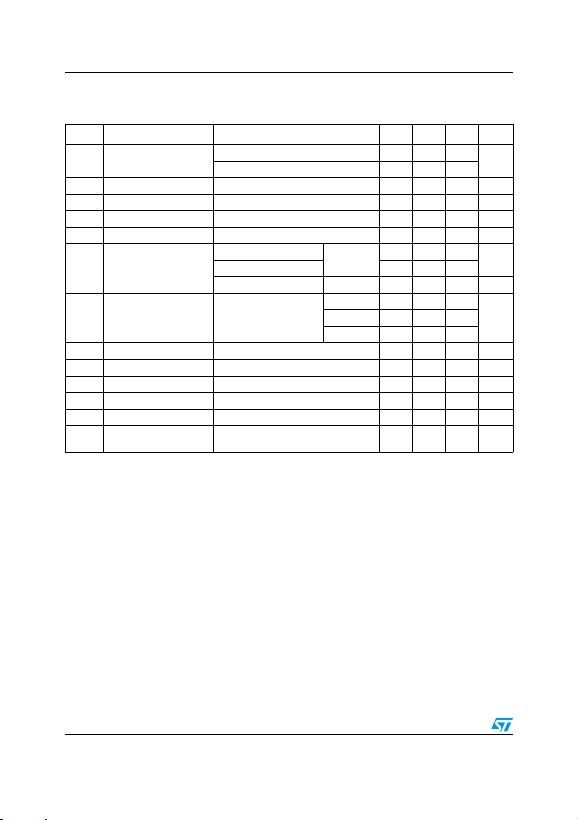Electrical characteristics LFxxAB, LFxxC
10/50 Doc ID 2574 Rev 25
Refer to the test circuits, TJ = 25 °C, CI = 0.1 µF, CO = 2.2 µF unless otherwise specified.
Table 6. Electrical characteristics for LF18C
Symbol Parameter Test conditions Min. Typ. Max. Unit
VOOutput voltage IO = 50 mA, VI = 3.5 V 1.764 1.8 1.836 V
IO = 50 mA, VI = 3.5 V, Ta = -25 to 85°C 1.728 1.872
VIOperating input voltage IO = 500 mA 3 16 V
IOOutput current limit 1 A
ΔVOLine regulation VI = 2.8 to 16 V, IO = 5 mA 2 12 mV
ΔVOLoad regulation VI = 3.3 V, IO = 5 to 500 mA 2 10 mV
IdQuiescent current
VI = 2.5 to 16V, IO = 0mA ON MODE 0.5 1 mA
VI = 3.1 to 16V, IO=500mA 12
VI = 6 V OFF MODE 50 100 µA
SVR Supply voltage rejection IO = 5 mA, VI = 3.5 ± 1 V
f = 120 Hz 82
dBf = 1 kHz 77
f = 10 kHz 60
eN Output noise voltage B = 10 Hz to 100 kHz 50 µV
VdDropout voltage IO = 200 mA 0.7 V
VIL Control input logic low Ta = -40 to 125°C 0.8 V
VIH Control input logic high Ta = -40 to 125°C 2 V
IIControl input current VI = 6 V, VC = 6 V 10 µA
CO
Output bypass
capacitance ESR = 0.1 to 10 Ω, IO = 0 to 500 mA 2 10 µF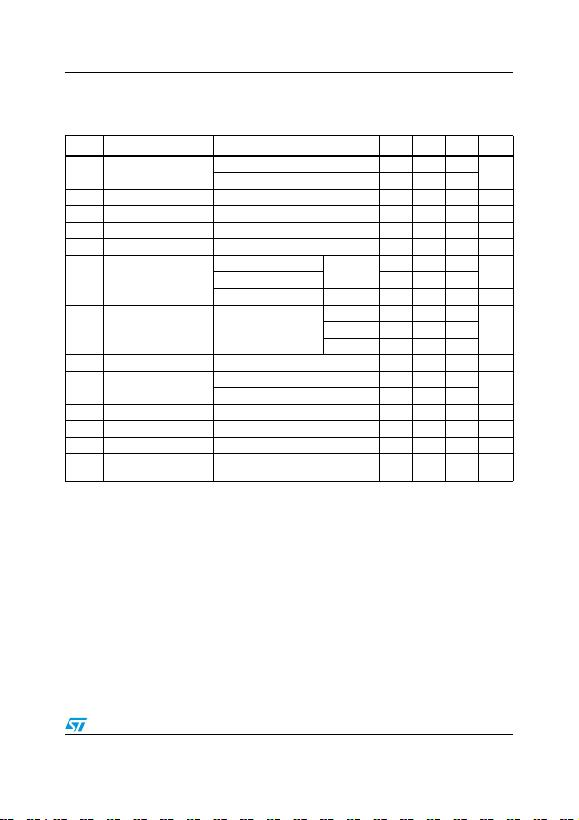LFxxAB, LFxxC Electrical characteristics
Doc ID 2574 Rev 25 11/50
Refer to the test circuits, TA = -40 to 125°C, CI = 0.1 µF, CO = 2.2 µF unless otherwise
specified.
Table 7. Electrical characteristics for LF18CDT-TRY (Automotive Grade)
Symbol Parameter Test conditions Min. Typ. Max. Unit
VOOutput voltage IO = 50 mA, VI = 3.5 V, Ta = 25°C 1.764 1.8 1.836 V
IO = 50 mA, VI = 3.5 V 1.713 1.887
VIOperating input voltage IO = 500 mA 3 16 V
IOOutput current limit Ta = 25°C 1 A
ΔVOLine regulation VI = 2.8 to 16 V, IO = 5 mA 2 15 mV
ΔVOLoad regulation VI = 3.3 V, IO = 5 to 500 mA 2 15 mV
IdQuiescent current
VI = 2.5 to 16V, IO = 0mA ON MODE 0.5 2 mA
VI = 3.1 to 16V, IO=500mA 12
VI = 6 V OFF MODE 50 120 µA
SVR Supply voltage rejection IO = 5 mA, VI = 3.5 ± 1 V
Ta = 25°C
f = 120 Hz 82
dBf = 1 kHz 77
f = 10 kHz 60
eN Output noise voltage B = 10 Hz to 100 kHz, Ta = 25°C 50 µV
VdDropout voltage IO = 200 mA 0.2 1.3 V
IO = 500 mA 0.4 1.3
VIL Control input logic low 0.8 V
VIH Control input logic high 2 V
IIControl input current VI = 6 V, VC = 6 V, Ta = 25°C 10 µA
CO
Output bypass
capacitance ESR = 0.1 to 10 Ω, IO = 0 to 500 mA 2 10 µF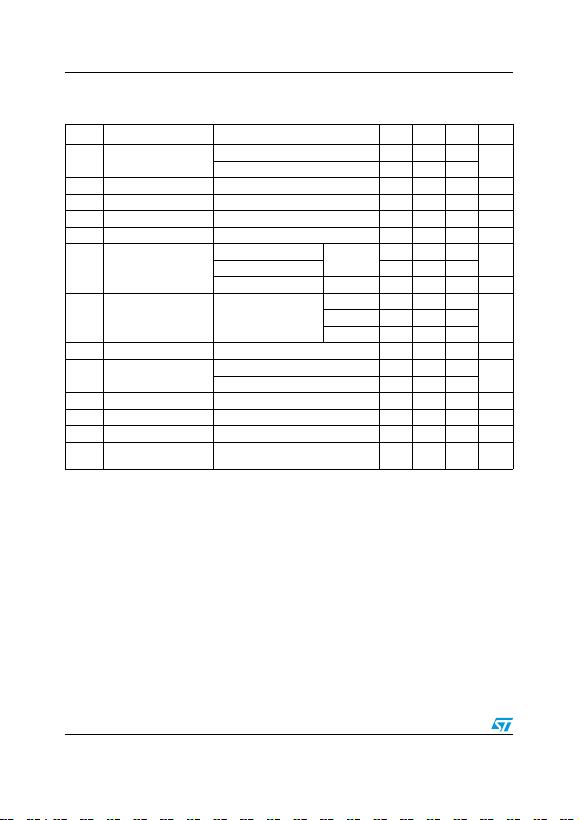Electrical characteristics LFxxAB, LFxxC
12/50 Doc ID 2574 Rev 25
Refer to the test circuits, TJ = 25 °C, CI = 0.1 µF, CO = 2.2 µF unless otherwise specified.
Table 8. Electrical characteristics for LF25AB
Symbol Parameter Test conditions Min. Typ. Max. Unit
VOOutput voltage IO = 50 mA, VI = 4.5 V 2.475 2.5 2.525 V
IO = 50 mA, VI = 4.5 V, Ta = -25 to 85°C 2.450 2.550
VIOperating input voltage IO = 500 mA 16 V
IOOutput current limit 1 A
ΔVOLine regulation VI = 3.5 to 16 V, IO = 5 mA 2 12 mV
ΔVOLoad regulation VI = 3.8 V, IO = 5 to 500 mA 2 12 mV
IdQuiescent current
VI = 3.5 to 16V, IO = 0mA ON MODE 0.5 1 mA
VI = 3.8 to 16V, IO=500mA 12
VI = 6 V OFF MODE 50 100 µA
SVR Supply voltage rejection IO = 5 mA, VI = 4.5 ± 1 V
f = 120 Hz 82
dBf = 1 kHz 77
f = 10 kHz 65
eN Output noise voltage B = 10 Hz to 100 kHz 50 µV
VdDropout voltage IO = 200 mA 0.2 0.35 V
IO = 500 mA 0.4 0.7
VIL Control input logic low Ta = -40 to 125°C 0.8 V
VIH Control input logic high Ta = -40 to 125°C 2 V
IIControl input current VI = 6 V, VC = 6 V 10 µA
CO
Output bypass
capacitance ESR = 0.1 to 10 Ω, IO = 0 to 500 mA 2 10 µF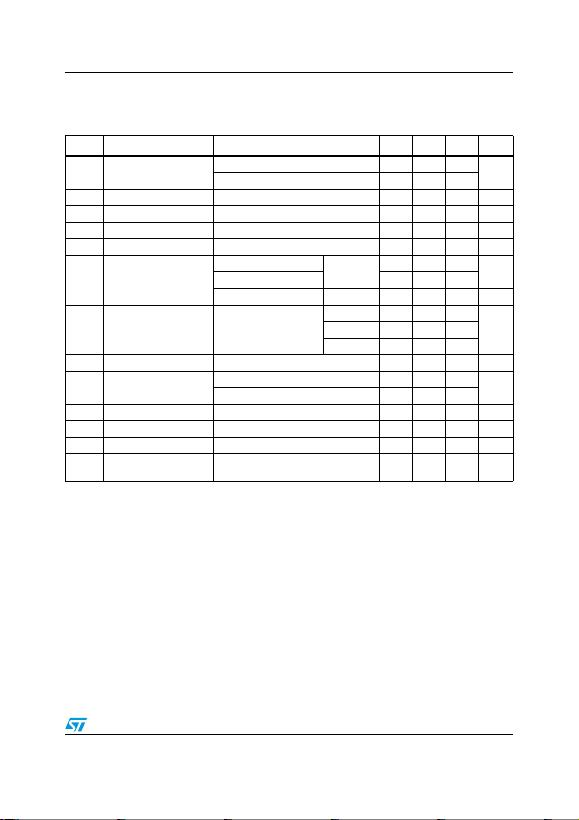LFxxAB, LFxxC Electrical characteristics
Doc ID 2574 Rev 25 13/50
Refer to the test circuits, TA = -40 to 125°C, CI = 0.1 µF, CO = 2.2 µF unless otherwise
specified.
Table 9. Electrical characteristics for LF25ABDT-TRY (Automotive Grade)
Symbol Parameter Test conditions Min. Typ. Max. Unit
VOOutput voltage IO = 50 mA, VI = 4.5 V, Ta = 25°C 2.475 2.5 2.525 V
IO = 50 mA, VI = 4.5 V 2.435 2.565
VIOperating input voltage IO = 500 mA 16 V
IOOutput current limit Ta = 25°C 1 A
ΔVOLine regulation VI = 3.5 to 16 V, IO = 5 mA 2 15 mV
ΔVOLoad regulation VI = 3.8 V, IO = 5 to 500 mA 2 15 mV
IdQuiescent current
VI = 3.5 to 16V, IO = 0mA ON MODE 0.5 2 mA
VI = 3.8 to 16V, IO=500mA 12
VI = 6 V OFF MODE 50 120 µA
SVR Supply voltage rejection IO = 5 mA, VI = 4.5 ± 1 V
Ta = 25°C
f = 120 Hz 82
dBf = 1 kHz 77
f = 10 kHz 65
eN Output noise voltage B = 10 Hz to 100 kHz, Ta = 25°C 50 µV
VdDropout voltage IO = 200 mA 0.2 1.3 V
IO = 500 mA 0.4 1.3
VIL Control input logic low 0.8 V
VIH Control input logic high 2 V
IIControl input current VI = 6 V, VC = 6 V, Ta = 25°C 10 µA
CO
Output bypass
capacitance ESR = 0.1 to 10 Ω, IO = 0 to 500 mA 2 10 µF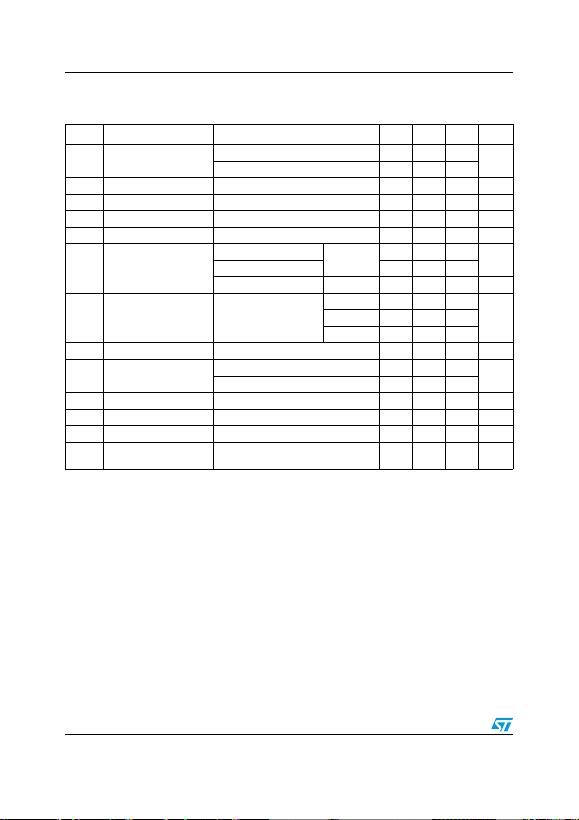Electrical characteristics LFxxAB, LFxxC
14/50 Doc ID 2574 Rev 25
Refer to the test circuits, TJ = 25 °C, CI = 0.1 µF, CO = 2.2 µF unless otherwise specified.
Table 10. Electrical characteristics for LF25C
Symbol Parameter Test conditions Min. Typ. Max. Unit
VOOutput voltage IO = 50 mA, VI = 4.5 V 2.45 2.5 2.55 V
IO = 50 mA, VI = 4.5 V, Ta = -25 to 85°C 2.4 2.6
VIOperating input voltage IO = 500 mA 16 V
IOOutput current limit 1 A
ΔVOLine regulation VI = 3.5 to 16 V, IO = 5 mA 2 12 mV
ΔVOLoad regulation VI = 3.8 V, IO = 5 to 500 mA 2 12 mV
IdQuiescent current
VI = 3.5 to 16V, IO = 0mA ON MODE 0.5 1 mA
VI = 3.8 to 16V, IO=500mA 12
VI = 6 V OFF MODE 50 100 µA
SVR Supply voltage rejection IO = 5 mA, VI = 4.5 ± 1 V
f = 120 Hz 82
dBf = 1 kHz 77
f = 10 kHz 65
eN Output noise voltage B = 10 Hz to 100 kHz 50 µV
VdDropout voltage IO = 200 mA 0.2 0.35 V
IO = 500 mA 0.4 0.7
VIL Control input logic low Ta = -40 to 125°C 0.8 V
VIH Control input logic high Ta = -40 to 125°C 2 V
IIControl input current VI = 6 V, VC = 6 V 10 µA
CO
Output bypass
capacitance ESR = 0.1 to 10 Ω, IO = 0 to 500 mA 2 10 µF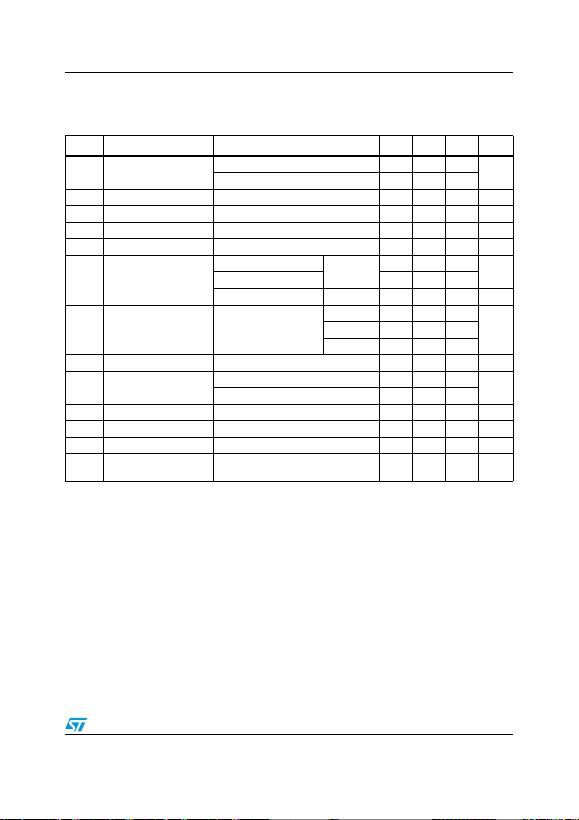LFxxAB, LFxxC Electrical characteristics
Doc ID 2574 Rev 25 15/50
Refer to the test circuits, TA = -40 to 125°C, CI = 0.1 µF, CO = 2.2 µF unless otherwise
specified.
Table 11. Electrical characteristics for LF25CDT-TRY (Automotive Grade)
Symbol Parameter Test conditions Min. Typ. Max. Unit
VOOutput voltage IO = 50 mA, VI = 4.5 V, Ta = 25°C 2.45 2.5 2.55 V
IO = 50 mA, VI = 4.5 V 2.385 2.615
VIOperating input voltage IO = 500 mA 16 V
IOOutput current limit Ta = 25°C 1 A
ΔVOLine regulation VI = 3.5 to 16 V, IO = 5 mA 2 15 mV
ΔVOLoad regulation VI = 3.8 V, IO = 5 to 500 mA 2 15 mV
IdQuiescent current
VI = 3.5 to 16V, IO = 0mA ON MODE 0.5 2 mA
VI = 3.8 to 16V, IO=500mA 12
VI = 6 V OFF MODE 50 120 µA
SVR Supply voltage rejection IO = 5 mA, VI = 4.5 ± 1 V
Ta = 25°C
f = 120 Hz 82
dBf = 1 kHz 77
f = 10 kHz 65
eN Output noise voltage B = 10 Hz to 100 kHz, Ta = 25°C 50 µV
VdDropout voltage IO = 200 mA 0.2 1.3 V
IO = 500 mA 0.4 1.3
VIL Control input logic low 0.8 V
VIH Control input logic high 2 V
IIControl input current VI = 6 V, VC = 6 V, Ta = 25°C 10 µA
CO
Output bypass
capacitance ESR = 0.1 to 10 Ω, IO = 0 to 500 mA 2 10 µF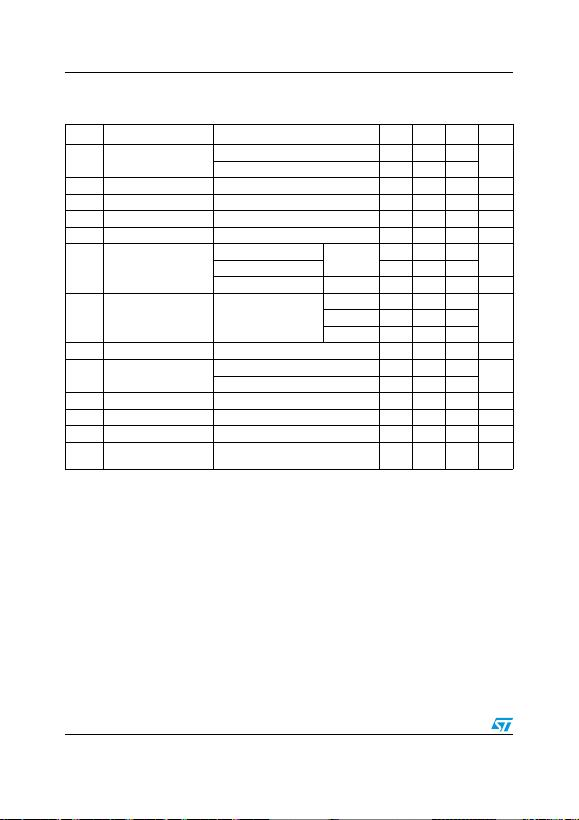Electrical characteristics LFxxAB, LFxxC
16/50 Doc ID 2574 Rev 25
Refer to the test circuits, TJ = 25 °C, CI = 0.1 µF, CO = 2.2 µF unless otherwise specified.
Table 12. Electrical characteristics for LF33AB
Symbol Parameter Test conditions Min. Typ. Max. Unit
VOOutput voltage IO = 50 mA, VI = 5.3 V 3.267 3.3 3.333 V
IO = 50 mA, VI = 5.3 V, Ta = -25 to 85°C 3.234 3.366
VIOperating input voltage IO = 500 mA 16 V
IOOutput current limit 1 A
ΔVOLine regulation VI = 4.3 to 16 V, IO = 5 mA 3 16 mV
ΔVOLoad regulation VI = 4.6 V, IO = 5 to 500 mA 3 16 mV
IdQuiescent current
VI = 4.3 to 16V, IO = 0mA ON MODE 0.5 1 mA
VI = 4.6 to 16V, IO=500mA 12
VI = 6 V OFF MODE 50 100 µA
SVR Supply voltage rejection IO = 5 mA, VI = 5.3 ± 1 V
f = 120 Hz 80
dBf = 1 kHz 75
f = 10 kHz 65
eN Output noise voltage B = 10 Hz to 100 kHz 50 µV
VdDropout voltage IO = 200 mA 0.2 0.35 V
IO = 500 mA 0.4 0.7
VIL Control input logic low Ta = -40 to 125°C 0.8 V
VIH Control input logic high Ta = -40 to 125°C 2 V
IIControl input current VI = 6 V, VC = 6 V 10 µA
CO
Output bypass
capacitance ESR = 0.1 to 10 Ω, IO = 0 to 500 mA 2 10 µF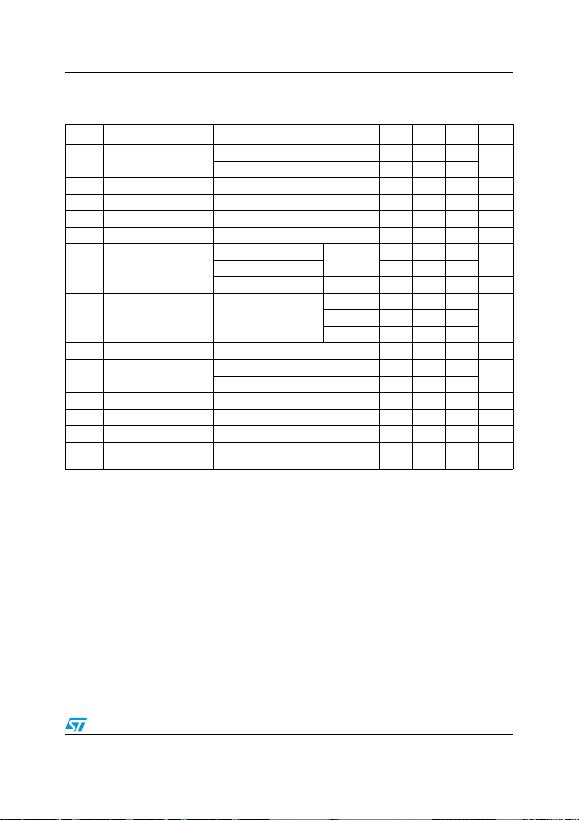LFxxAB, LFxxC Electrical characteristics
Doc ID 2574 Rev 25 17/50
Refer to the test circuits, TJ = 25 °C, CI = 0.1 µF, CO = 2.2 µF unless otherwise specified.
Table 13. Electrical characteristics for LF33C
Symbol Parameter Test conditions Min. Typ. Max. Unit
VOOutput voltage IO = 50 mA, VI = 5.3 V 3.234 3.3 3.366 V
IO = 50 mA, VI = 5.3 V, Ta = -25 to 85°C 3.168 3.432
VIOperating input voltage IO = 500 mA 16 V
IOOutput current limit 1 A
ΔVOLine regulation VI = 4.3 to 16 V, IO = 5 mA 3 16 mV
ΔVOLoad regulation VI = 4.6 V, IO = 5 to 500 mA 3 16 mV
IdQuiescent current
VI = 4.3 to 16V, IO = 0mA ON MODE 0.5 1 mA
VI = 4.6 to 16V, IO=500mA 12
VI = 6 V OFF MODE 50 100 µA
SVR Supply voltage rejection IO = 5 mA, VI = 5.3 ± 1 V
f = 120 Hz 80
dBf = 1 kHz 75
f = 10 kHz 65
eN Output noise voltage B = 10 Hz to 100 kHz 50 µV
VdDropout voltage IO = 200 mA 0.2 0.35 V
IO = 500 mA 0.4 0.7
VIL Control input logic low Ta = -40 to 125°C 0.8 V
VIH Control input logic high Ta = -40 to 125°C 2 V
IIControl input current VI = 6 V, VC = 6 V 10 µA
CO
Output bypass
capacitance ESR = 0.1 to 10 Ω, IO = 0 to 500 mA 2 10 µF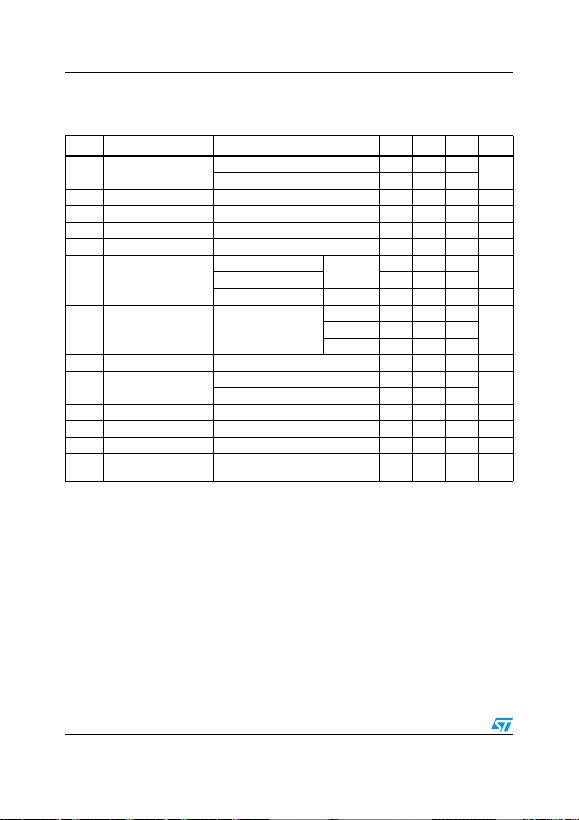Electrical characteristics LFxxAB, LFxxC
18/50 Doc ID 2574 Rev 25
Refer to the test circuits, TA = -40 to 125°C, CI = 0.1 µF, CO = 2.2 µF unless otherwise
specified.
Table 14. Electrical characteristics for LF33CDT-TRY and LF33CPT-TRY (Automotive Grade)
Symbol Parameter Test conditions Min. Typ. Max. Unit
VOOutput voltage IO = 50 mA, VI = 5.3 V, Ta = 25°C 3.234 3.3 3.366 V
IO = 50 mA, VI = 5.3 V, 3.153 3.447
VIOperating input voltage IO = 500 mA 16 V
IOOutput current limit Ta = 25°C 1 A
ΔVOLine regulation VI = 4.3 to 16 V, IO = 5 mA 3 19 mV
ΔVOLoad regulation VI = 4.6 V, IO = 5 to 500 mA 3 19 mV
IdQuiescent current
VI = 4.3 to 16V, IO = 0mA ON MODE 0.5 2 mA
VI = 4.6 to 16V, IO=500mA 12
VI = 6 V OFF MODE 50 120 µA
SVR Supply voltage rejection IO = 5 mA, VI = 5.3 ± 1 V
Ta = 25°C
f = 120 Hz 80
dBf = 1 kHz 75
f = 10 kHz 65
eN Output noise voltage B = 10 Hz to 100 KHz, Ta = 25°C 50 µV
VdDropout voltage IO = 200 mA 0.2 1.3 V
IO = 500 mA 0.4 1.3
VIL Control input logic low 0.8 V
VIH Control input logic high 2 V
IIControl input current VI = 6 V, VC = 6 V, Ta = 25°C 10 µA
CO
Output bypass
capacitance ESR = 0.1 to 10 Ω, IO = 0 to 500 mA 2 10 µFLFxxAB, LFxxC Electrical characteristics
Doc ID 2574 Rev 25 19/50
Refer to the test circuits, TJ = 25 °C, CI = 0.1 µF, CO = 2.2 µF unless otherwise specified.
Table 15. Electrical characteristics for LF50AB
Symbol Parameter Test conditions Min. Typ. Max. Unit
VOOutput voltage IO = 50 mA, VI = 7 V 4.95 5 5.05 V
IO = 50 mA, VI = 7 V, Ta = -25 to 85°C 4.9 5.1
VIOperating input voltage IO = 500 mA 16 V
IOOutput current limit 1 A
ΔVOLine regulation VI = 6 to 16 V, IO = 5 mA 5 25 mV
ΔVOLoad regulation VI = 6.3 V, IO = 5 to 500 mA 5 25 mV
IdQuiescent current
VI = 6 to 16V, IO = 0mA ON MODE 0.5 1 mA
VI = 6.3 to 16V, IO=500mA 12
VI = 6 V OFF MODE 50 100 µA
SVR Supply voltage rejection IO = 5 mA, VI = 7 ± 1 V
f = 120 Hz 76
dBf = 1 kHz 71
f = 10 kHz 60
eN Output noise voltage B = 10 Hz to 100 kHz 50 µV
VdDropout voltage IO = 200 mA 0.2 0.35 V
IO = 500 mA 0.4 0.7
VIL Control input logic low Ta = -40 to 125°C 0.8 V
VIH Control input logic high Ta = -40 to 125°C 2 V
IIControl input current VI = 6 V, VC = 6 V 10 µA
CO
Output bypass
capacitance ESR = 0.1 to 10 Ω, IO = 0 to 500 mA 2 10 µFElectrical characteristics LFxxAB, LFxxC
20/50 Doc ID 2574 Rev 25
Refer to the test circuits, TA = -40 to 125°C, CI = 0.1 µF, CO = 2.2 µF unless otherwise
specified.
Table 16. Electrical characteristics for LF50ABDT-TRY (Automotive Grade)
Symbol Parameter Test conditions Min. Typ. Max. Unit
VOOutput voltage IO = 50 mA, VI = 7 V, Ta = 25°C 4.95 5 5.05 V
IO = 50 mA, VI = 7 V 4.885 5.115
VIOperating input voltage IO = 500 mA 16 V
IOOutput current limit Ta = 25°C 1 A
ΔVOLine regulation VI = 6 to 16 V, IO = 5 mA 5 28 mV
ΔVOLoad regulation VI = 6.3 V, IO = 5 to 500 mA 5 28 mV
IdQuiescent current
VI = 6 to 16V, IO = 0mA ON MODE 0.5 2 mA
VI = 6.3 to 16V, IO=500mA 12
VI = 6 V OFF MODE 50 120 µA
SVR Supply voltage rejection IO = 5 mA, VI = 7 ± 1 V
Ta = 25°C
f = 120 Hz 76
dBf = 1 kHz 71
f = 10 kHz 60
eN Output noise voltage B = 10 Hz to 100 kHz, Ta = 25°C 50 µV
VdDropout voltage IO = 200 mA 0.2 1.3 V
IO = 500 mA 0.4 1.3
VIL Control input logic low 0.8 V
VIH Control input logic high 2 V
IIControl input current VI = 6 V, VC = 6 V, Ta = 25°C 10 µA
CO
Output bypass
capacitance ESR = 0.1 to 10 Ω, IO = 0 to 500 mA 2 10 µFLFxxAB, LFxxC Electrical characteristics
Doc ID 2574 Rev 25 21/50
Refer to the test circuits, TJ = 25 °C, CI = 0.1 µF, CO = 2.2 µF unless otherwise specified.
Table 17. Electrical characteristics for LF50C
Symbol Parameter Test conditions Min. Typ. Max. Unit
VOOutput voltage IO = 50 mA, VI = 7 V 4.9 5 5.1 V
IO = 50 mA, VI = 7 V, Ta = -25 to 85°C 4.8 5.2
VIOperating input voltage IO = 500 mA 16 V
IOOutput current limit 1 A
ΔVOLine regulation VI = 6 to 16 V, IO